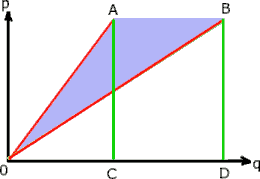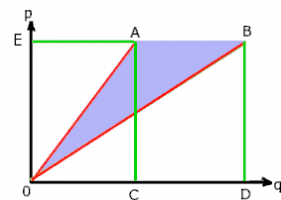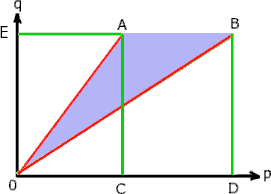# Number Theory

#### Jomo

##### Elite Member
20 <= p/q <=21

Pick some small values for q and see if you can find a pattern.

For example, if q=1, then p can be 20 or 21.
If q=2, then p = 40, 41 or 42
If q=3, then p = 62 or p=63

#### Otis

##### Elite Member
20 <= p/q <=21
Hi Jomo (stupid phone keeps changing yer name to Joni). Had you intended to work with

20 <= 50p/q <= 21

or are you using a different example for illustration purposes?

In the given exercise, q cannot be 1,2,3,4,6,7,8,9,11,13,14,16,18,19,... because p values would not be Integers.

I'd started by writing

40/100 <= p/q <= 42/100

and thinking about how many p/q would equal 0.4 (i.e., equivalent fractions -- q is multiple of 5), and then I'd wondered if there might be a way to determine how many wouldn't.

(There are no additional fractions equivalent to 21/50, so the only p/q with q=50 would be 20/50 and 21/50.)

Manually checking with low q values, it appeared that the number (n) of p/q without a terminating decimal form appearing after q being a multiple of 5 might be increasing like this:

q, n
5, 0
10, 1
15, 1
20, 2
25, 2
30, 3
35, 3
etc

That would help with counting all p's and q's, but we don't want to include any repeats, so my approach would need a lot of manual checking.#### Otis

##### Elite Member
I have tried transposing the variables•Cubist

#### Cubist

##### Senior Member
@Otis is correct, in order to guide you we need to know what strategy your teacher expects you to use. Therefore please show some of your work. In the absence of this, here are a couple of extra ideas...

I'm quite a visual thinker, so personally I'd think about a method to count the number of integer (q, p) grid points in the light blue region of the following graph (including any grid points that lie ON the red lines).OR, actually, I'd be thinking of writing a computer program to count the solutions since it's such a small set of q values!

Last edited:
•Subhotosh Khan

#### Otis

##### Elite Member
writing a computer program to count
That would be simplest, but I'm hoping the OP will teach me something from number theory.•Jomo

#### Jomo

##### Elite Member
Hi Jomo (stupid phone keeps changing yer name to Joni). Had you intended to work with

20 <= 50p/q <= 21Yes, I did mean to write 20 <= 50p/q <= 21

#### Jomo

##### Elite Member
@Otis is correct, in order to guide you we need to know what strategy your teacher expects you to use. Therefore please show some of your work. In the absence of this, here are a couple of extra ideas...

I'm quite a visual thinker, so personally I'd think about a method to count the number of integer (q, p) grid points in the light blue region of the following graph (including any grid points that lie ON the red lines).

View attachment 28892

OR, actually, I'd be thinking of writing a computer program to count the solutions since it's such a small set of q values!
Computer program sometimes get in the way of real mathematics.

#### Cubist

##### Senior Member
That would be simplest, but I'm hoping the OP will teach me something from number theory.Computer program sometimes get in the way of real mathematics.
I know where you're both coming from, and (partly) agree with you both. It does seem very unlikely that @AdkAdi was being asked to write a computer algorithm to solve this. I was trying to illustrate that there are different approaches, and without seeing any work from OP how are we supposed to provide relevant help?

Last edited:
•Subhotosh Khan

#### Cubist

##### Senior Member
...I'd think about a method to count the number of integer (q, p) grid points in the light blue region of the following graph (including any grid points that lie ON the red lines).
Here's an improved image and an extra hint for my first proposed method...HINT: Can you see that the number of grid intersection points in 0EAC will be double the number of grid intersection points in 0AC (but you'll need to be careful and take into account the grid intersection points that lie along the outer lines of the shapes).
NOTE: I have not worked this out to an end result, but I think this method could be used to yield a closed form solution for any upper-limit of q (not just 99)

#### mmm4444bot

##### Super Moderator
Staff member
without seeing any work from OP how are we supposed to provide relevant help?
One way is to ask the OP questions and wait.

Another way is to not wait but guess (including a proviso, perhaps).

On the other hand, many replies here are written for a general audience (not concerned with relevance for the OP per se, as long as there's relevance for some students in what they say).

I don't see any issues with your suggestions.#### Otis

##### Elite Member
Are you taking a number theory class? If not, then what class are you in and what has been discussed recently?##### New member
Are you taking a number theory class? If not, then what class are you in and what has been discussed recently?I am preparing for a Regional math Olympiad competition and for that I need to have a good amount of grip on Number Theory.

#### Otis

##### Elite Member
Thank you for providing context. I'm not the tutor to lead you toward discoveries in this subject, but I'll be interested to follow any discussion from those who are. Looking at patterns (by writing out some possibilities) and experimenting with what I already know is all I can try on paper. I'm thinking there must be insight to be gained from the subject -- some theorem or concept for simplifying the counts.#### Otis

##### Elite Member
Are you allowed to use any technology, in the competition?

[imath]\;[/imath]

##### New member
Are you allowed to use any technology, in the competition?

[imath]\;[/imath]
No not at all

•Otis

#### Otis

##### Elite Member
No not at all
Then our choices are currently limited (for quick methods using paper & pencil) until someone posts what we're missing.

Have you tried thinking about a simpler form, similar to the given situation?#### Cubist

##### Senior Member
Obtaining the answer using my suggested method was more complicated than I thought. There must be an easier method. But I'll show you my work anyway (I also verified the answer via computer)

20q ≤ 50p ≤ 21q

The LHS inequality is shown as line 0A and RHS as line 0B in the following graph...#intersections in shaded area 0AB (including all grid intersections that occur ON the outer lines - subsequently referred to as "outers")
= 0AC (with all outers) + ABDC (with all outers except AC) - 0BD (with all outers except 0B)

To calculate the number of grid intersection points within triangle 0AC, turn the triangle into rectangle 0EAC, subtract the number of intersections that occur on the diagonal 0A, and then divide by two, and finally add back the number of diagonal intersection points. For this to work, the outer edges of the rectangle must align with the grid intersection points. Therefore increase the range of q to 0≤q≤100 (which yields integer coordinates for A,B,C and D), and then at the end subtract the results for q=0 and q=100 to obtain the desired range of 1≤q≤99

At point E q=100
At points A and C, p=100*20/50 = 40
At points B and D, p=100*21/50 = 42

OAC (with all outers)
The number of grid point intersections on line 0A is Ia=21. The first few are (0,0) (2,5) (4,10) (6,15)...(40,100) therefore the total number is (100/5)+1
Number of intersections within OAC is ((40+1)*(100+1) - Ia )/2 + Ia = 2081

ABDC (with all outers except AC)
(42-40) * (100+1) = 202

OBD (with all outers except on line 0B)
The number of grid point intersections on line 0A is Ib=3. These are (0,0) (21,50) (42,100)
Number of intersections within ODB is ((42+1)*(100+1) - Ib)/2 = 2170

INTERIM RESULT
0AC + ABDC - 0BD
= 2081 + 202 - 2170
= 113

FINAL RESULT
Remove intersections for q=0, and q=100. When q=0 we have 0 ≤ p ≤ 0 therefore one option at (0,0)
and when q=100 we have 40 ≤ p≤ 42 therefore 3 options at (40,100) (41,100) (42,100)

Removing these gives number of (p,q)
= 113 - (1+3)
= 109

Last edited: